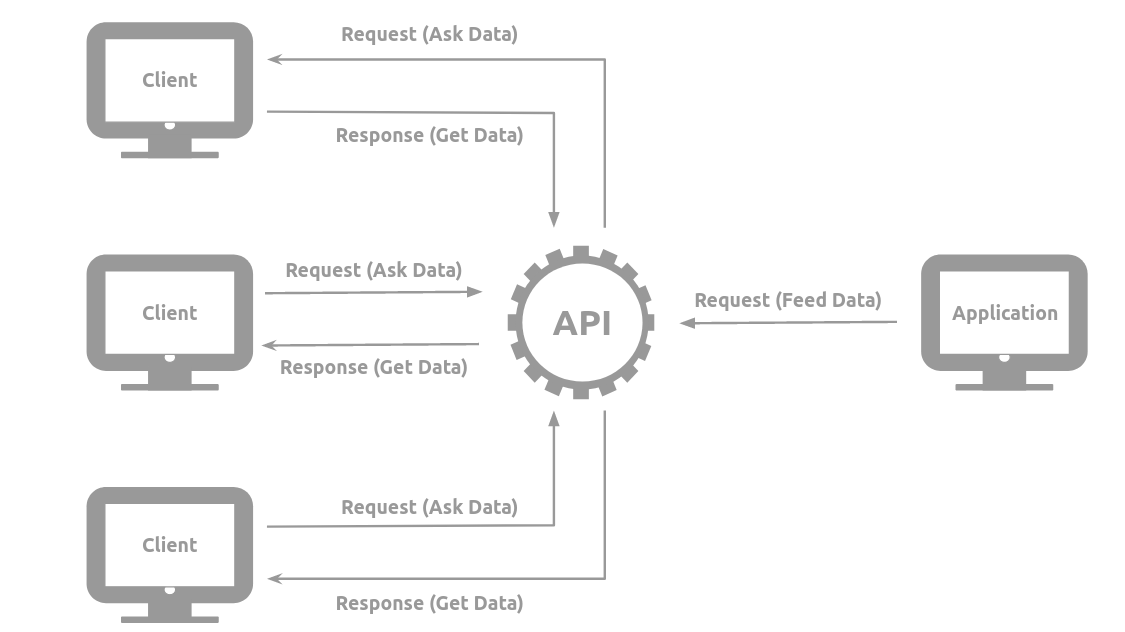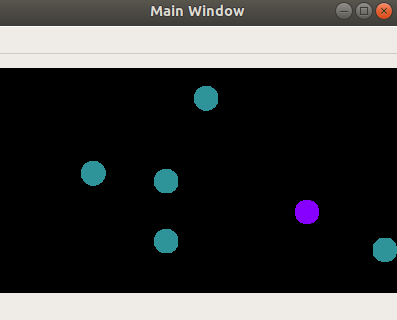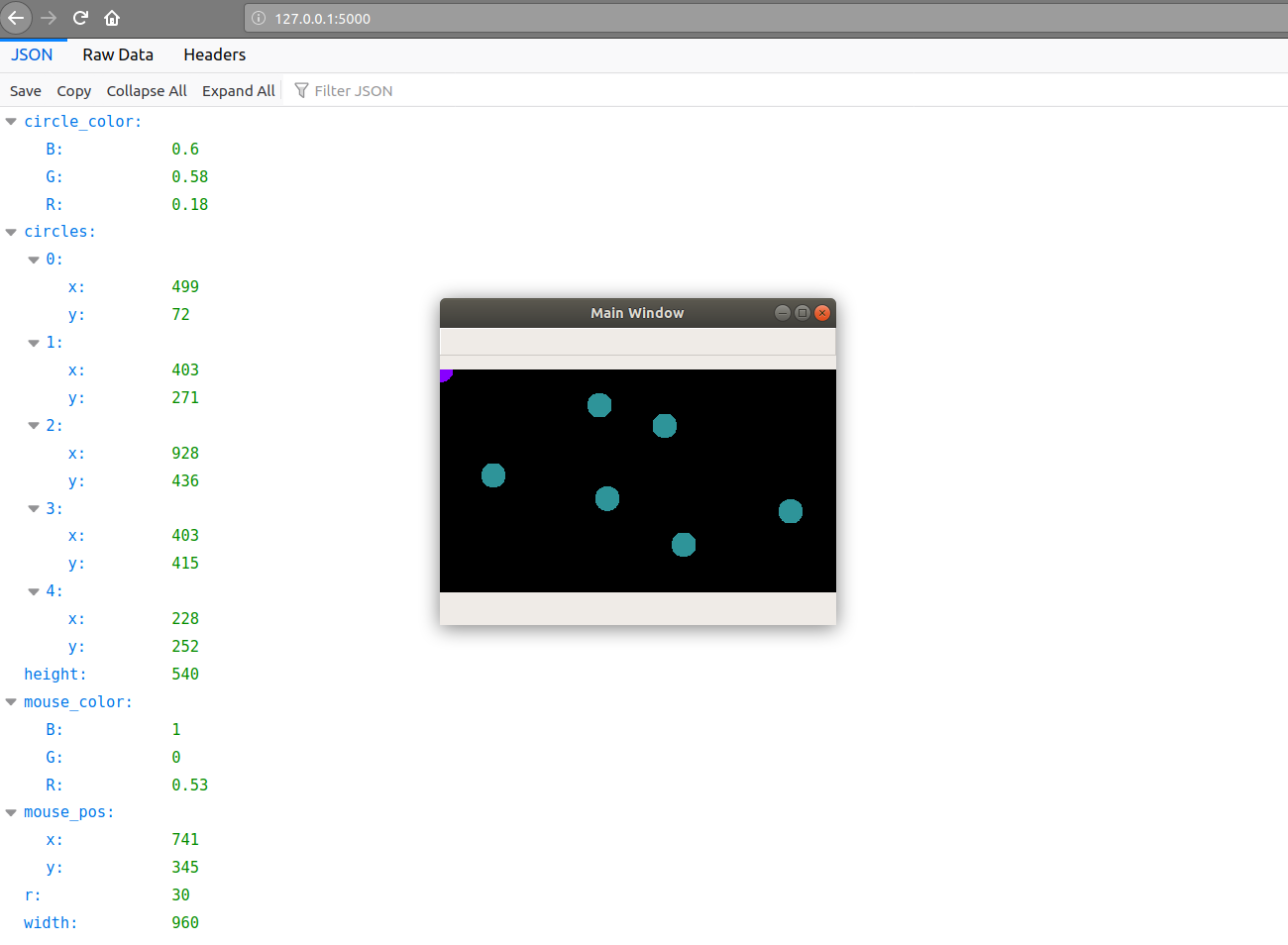# How to design a real time API with Python using Flask ?

Tutorial

Design a python API. From the creation of the application, to the client through the interface.

Informations
• axel.thevenot@edu.devinci.fr
• 06 24 98 20 33
Dates
• Creation: 02/23/2020
• Update: 03/31/2020
axel_thevenotbillard-interactif

Application Programming Interface

Before briefly describing what an Application Programming Interface is (API) I would like to clarify what an interface is.Now in IT, the API can be summarized as an interface that allows applications to communicate and exchange data or services. An API is therefore an interface between clients and applications.

Requirements

In this tutorial we will design an API, its application and client side use with python using Flask. So we need to install some libraries.

```pip install numpy opencv-python flask flask-cors
```

To make it simple and visual, I've prepared a little python script. It's only a class to open an interactive OpenCV window. We will be able to move our mouse in this window and tag points with a left mouse click. This will be our application that we will place in a file titled window.py.

```import cv2
import numpy as np

class Window:
def __init__(self, width, height, r, mouse_color, circle_color, main=True):
# get a frame
self.frame = np.zeros((height, width, 3))

# get the circles attributes
self.r = r
self.mouse_color = mouse_color
self.circle_color = circle_color

# create a variable to store the circles and the mouse position
self.mouse_pos = (0, 0)
self.circles = []

# create a window interactive if asked
if main:
cv2.namedWindow('Main Window', cv2.WINDOW_NORMAL)
cv2.setMouseCallback('Main Window', self.mouse_event)
else:
cv2.namedWindow('Client Window', cv2.WINDOW_NORMAL)

def mouse_event(self, event, x, y, flags, param):
# actualize the mouse position
self.mouse_pos = (x, y)
# add circle if left mouse button clicked (when released)
if event == cv2.EVENT_LBUTTONUP:
self.circles.append(self.mouse_pos)

def update_frame(self):
# reset the frame
self.frame = self.frame * 0
# draw the circles stored and the mouse position in the frame
for circle in self.circles:
cv2.circle(self.frame, circle, self.r, self.circle_color, -1)
cv2.circle(self.frame, self.mouse_pos, self.r, self.mouse_color, -1)

```API

So we can start designing our API. This one, for simplification reasons, will be hosted in localhost (or 127.0.0.1) on port 5000. You are free to modify this setting as you wish. We will integrate the python code of the API in a file titled API.py.

```import threading

# create a variable to store the data
data = None
# make cross-origin AJAX possible
CORS(app)

# create a method to send the data to the API when requested
@app.route("/")
def send_data():
# convert into JSON format first
return jsonify(data)

def start(host, port):
# get the host and the port as keywords attributes for app.run()
app_kwargs = {'host':host, 'port':port}
# run the app on a thread
```

First, we have a data variable. It is this variable that will then define what the API's clients will have access to. To send this data to the chosen address (https://127.0.0.1:5000/), we create the send_data() function that will send the data stored in the data variable at each request. This data will be converted beforehand into JSON format. The JSON format is the format that is the most used in APIs. By analogy with python, a JSON object is a kind of dictionary.

Finally it will be necessary to be able to launch the API. We have created the start() method which launches the application on a thread. This allows not to disturb the python program. Indeed this one would have been "stopped" at this line until the application is interrupted.

I did not mention the CORS(app) line. CORS, for Cross Origin Resource Sharing, gives access to the application in all cases. This extension of Flask allows to formulate AJAX requests.

Our API is technically ready. We still have to define the application and the data that will be accessible to customers.

First of all, to convert our data into JSON format, we will need to convert them into python dictionary format beforehand. In the window.py file, in the Window class we can add the following method that will be used to return all the data of our structured object in dictionary form.

```    def to_dict(self):
d = {}
d['width'] = self.frame.shape
d['height'] = self.frame.shape
d['r'] = self.r
b, g, r = self.mouse_color
d['mouse_color'] = {'B':b, 'G':g, 'R':r}
b, g, r = self.circle_color
d['circle_color'] = {'B':b, 'G':g, 'R':r}
d['mouse_pos'] = {'x': self.mouse_pos, 'y': self.mouse_pos}
d['circles'] = [{'x': x, 'y': y} for x, y in self.circles]
return d

```

Application

So let's create the application in the main.py file that will exchange its data with future customers. You will be able to change the variables in capital letters.

```import cv2
import numpy as np

import API as api
from window import Window

# set the host and the port (here in localhost)
HOST, PORT = '127.0.0.1', 5000
# set the image dimension
W, H = 960, 540
# set the radius of the circles and their color
R = 30
MOUSE_COLOR = (1, 0, 0.53)
CIRLCE_COLOR = (0.6, 0.58, 0.18)

if __name__ == '__main__':
# create an interactive window
interactive_window = Window(W, H, R, MOUSE_COLOR, CIRLCE_COLOR, main=True)
# start the API
api.start(host=HOST, port=PORT)

while True:

# update the interactive window frame
interactive_window.update_frame()
# update the data to send to the API
api.data = interactive_window.to_dict()
# display the interactive window
cv2.imshow('Main Window', interactive_window.frame)
# exit the program by pressing 'q' key
if cv2.waitKey(1) & 0xff == ord('q'):
break

cv2.destroyAllWindows()

```

If everything is clear so far then the application script should not be a problem for you. First we initialize a window with OpenCV and at the same time we start the API. Then in real time, the window will be interactive with the mouse and its data (its descriptive python dictionary) will be sent to the API in JSON format. If you run this script and connect to a browser at the defined address (here http://127.0.0.1:5000/) you will be able to view the data in JSON format.Our interface is now created. So it is now possible for a client to process and retrieve these data and recreate the application window in real time. In the window.py file we can therefore add this last little method to build an object from a dictionary (as opposed to the to_dict() function).

```def from_dict(d):
# get all the attributes from the dictionary
width = d['width']
height = d['height']
r = d['r']
d_col = d['mouse_color']
mouse_color = (d_col['B'], d_col['G'], d_col['R'])
d_col = d['circle_color']
circle_color = (d_col['B'], d_col['G'], d_col['R'])
mouse_pos = (d['mouse_pos']['x'], d['mouse_pos']['y'])
# create the window and add the other missing attributes from __init__()
circles = [(d_circle['x'], d_circle['y']) for d_circle in d['circles']]
window = Window(width, height, r, mouse_color, circle_color, main=False)
window.mouse_pos = mouse_pos
window.circles = circles
return window

```

Client

Finally, in a client.py file we will recover the data from the url by converting it from JSON format to a python dictionary. Then we rebuild the window and finally we display it.

```import json
import urllib.request as urllib

import cv2

from window import from_dict

HOST, PORT = '127.0.0.1', 5000
# get the url as a string
url = f'http://{HOST}:{PORT}/'

def get_data_to_dict(url):
# open url
oper_url = urllib.urlopen(url)
if(oper_url.getcode()==200):
# get data and convert it to dictionary
else:
print("Error receiving data", oper_url.getcode())
return None

if __name__ == '__main__':
while True:
# get the data from the API as a dictionary
d = get_data_to_dict(url)
# if exists, recreate the window
if d is not None:
window_from_api = from_dict(d)
window_from_api.update_frame()
cv2.imshow('Client Window', window_from_api.frame)
# exit the program by pressing 'q' key
if cv2.waitKey(1) & 0xff == ord('q'):
break
```

We can run the python program main.py right now and then as many clients.py as we want.

Source